Courses

# Logic Gates MSQ

## 10 Questions MCQ Test Topic wise Tests for IIT JAM Physics | Logic Gates MSQ

Description
This mock test of Logic Gates MSQ for Physics helps you for every Physics entrance exam. This contains 10 Multiple Choice Questions for Physics Logic Gates MSQ (mcq) to study with solutions a complete question bank. The solved questions answers in this Logic Gates MSQ quiz give you a good mix of easy questions and tough questions. Physics students definitely take this Logic Gates MSQ exercise for a better result in the exam. You can find other Logic Gates MSQ extra questions, long questions & short questions for Physics on EduRev as well by searching above.
QUESTION: 1

### The output of which of the following logic gate is high when one or both of the input is low :

Solution:

In digital electronics, a NAND gate (NOT-AND) is a logic gate which produces an output which is false only if all its inputs are true; thus its output is complement to that of an AND gate. A LOW (0) output results only if all the inputs to the gate are HIGH (1); if any input is LOW (0), a HIGH (1) output results.

QUESTION: 2

### Which of the following gate can be build using a single transistor?

Solution:

Solution :- A transistor can be used as a switch. That is, when base is low collector is high (input zero, output one) and base is high collector is low (input 1, output 0).

*Multiple options can be correct
QUESTION: 3

### If the input to a NOT gate is A and the output is X, then :

Solution:

The correct answer is: X =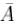*Multiple options can be correct
QUESTION: 4

How many of the inputs of a 4 input AND gate must be HIGH in order for the output of the logic gate to go HIGH?

Solution:

The correct answer is: all 4

*Multiple options can be correct
QUESTION: 5

The output of a logic gate is high when all its inputs are at logic 0. The gate can be :

Solution:

The correct answers are: NOR, XNOR, NAND

*Multiple options can be correct
QUESTION: 6

When simplified with boolean algebra, the expression (x + y)(x + z) simplies to :

Solution:

The correct answer is: x + yz

*Multiple options can be correct
QUESTION: 7

Which gates are required to build an exclusive OR (XOR) gate?

Solution:

The correct answers are: OR, AND, NOT

*Multiple options can be correct
QUESTION: 8

The simplification for the boolean expression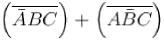is equivalent to :

Solution:

The correct answers are: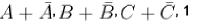*Multiple options can be correct
QUESTION: 9

The boolean expression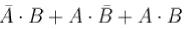is equivalent to :

Solution:

The correct answers are: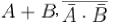*Multiple options can be correct
QUESTION: 10

Choose the correct options :

Solution:

The correct answers are: The boolean expression of an AND gate followed by an inverter is same as the boolean expression for a NAND gate., The boolean expression for an OR gate followed by a NOT gate is same as that for a NOR gate, No two basic logic gates have the same boolean expression